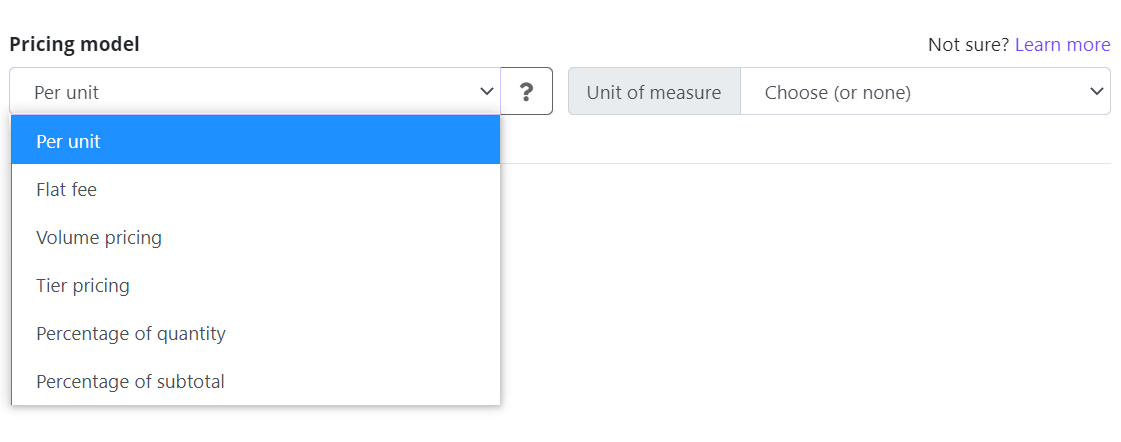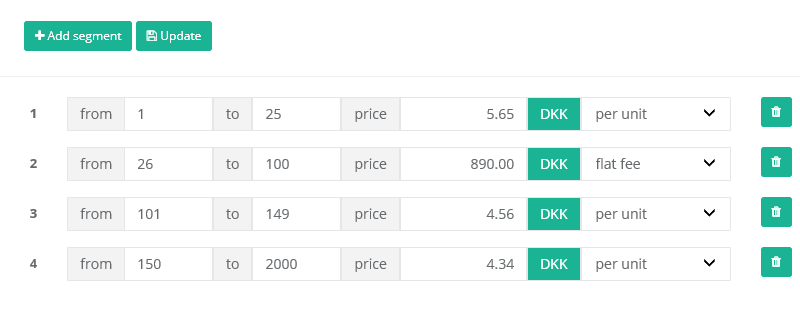# Pricing models

Upodi provides support for various monetization models. A monetization model consists of a pricing model and a charge type.

Upodi supports:

• Flat fee
• Per unit
• Tiered pricing
• Volume pricing
• Percentage of quantity
• Percentage of subtotal

The following outlines the different use.### 🚧Please be aware

Due to support of underlying finance systems (ERP), Upodi will handle all decimals when calculating tier and volume pricing, by supplementing quantity with 1 x the calculated price and adding the full quantity of this to the invoice line. ERP systems often calculate pricing of amount x quantity price, rendering the full amount wrong if decimals becomes larger than 2.

### Flat-fee

A flat fee pricing model bills a subscription a fixed amount in the term of the subscription. Flat fee pricing models ignore the quantity of the subscription charge, however, a subscription charge quantity of 0 will ignore the fee in a billing cycle.

Flat fee pricing models are usually used for sign-up fees, cancellation fees and based level subscription fees.

``````flatfee or 0
``````

### Per-unit

A per-unit pricing model, multiplies the per-unit amount with the quantity of a subscription charge. The following formula is used

``````quantity * per-unit price
``````

Per unit fees are often used in SaaS subscription plans (per user) or mobile data plans (per gb data).

### Tiered pricing

A tiered pricing model offers a flexible billing based on the quantity amount of a subscription. A tiered pricing model is calculated based on the quantity tier and type of that tier multiplying each tier of the full quantity.Each tier can be charged by per-unit pricing level (see above), or a Flat fee level (see above).

#### Example:

A tiered pricing model is calculated by the sum of all tiers matching the volume. Let's try to define this by looking at a sample table.

Tier

Pricing level

Pricing

Range

A

Per-unit

\$20 per unit

1-100

B

Per-unit

\$15 per unit

101-200

C

Per-unit

\$10 per unit

201-300

A tiered pricing model for a quantity of 130, will sample calculation of tier A plus tier B but not tier C. The calculation is done as follows:

``````Tier A = 100 * \$20 (per unit) = \$2.000
+
Tier B = 30 (remainder) * \$15 = \$450
================
Totals = \$2.450
``````

### Volume pricing

A volume pricing is often called a "discount ladder". A volume pricing models looks for a given tier of the volume pricing model, and calculates the total quantity of this tier.

#### Example

Using the sample table from above (tiered pricing), a volume pricing model, calculated with a quantity of 130, is done as follows. 130 matches tier B:

``````Tier B = 130 * \$15 = \$1.950
================
Totals = \$1.950
``````

### Percentage of quantity

A percentage of quantity charge will calculate its price based on the quantity collected by either metered calculation or quantity of the charge.

#### Example

A charge have the quantity of 1.250, and the pricing model of percentage of quantity is set to 5% with a currency of EUR. The calculation of pricing is done as follows:

``````Totals = 1.250 * 5% = 62.5 EUR
``````

### Percentage of subtotal

There can be only oné (1) 'percentage of subtotal' charge per product plan. In addition, this charge will be the last charge to proces.

The charge is calculated by sub-calculating the prior charges and then applying a percentage of subtotal as the last line.

#### Example

3 charges exist on the product plan, the first a flat fee charge, then a per unit charge and finally the percentage of subtotal of 5%.

``````Charge 1 = \$400
Charge 2 = 10 * \$15 = \$150
Charge 3 = 5% * (\$150 + \$400) = \$27.5
================
Totals = \$577.5
``````

## Questions?

We're always happy to help with code or other questions you might have! Search our documentation or contact helpdesk. You can also chat live with us using the Intercom icon.